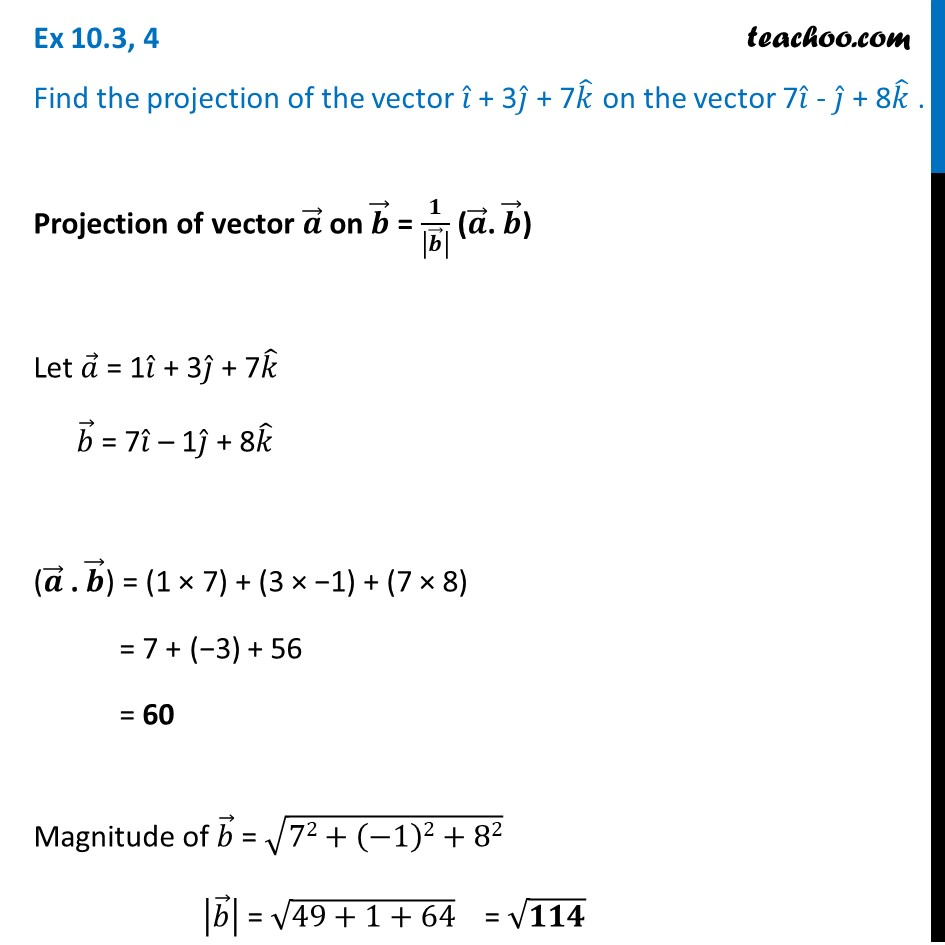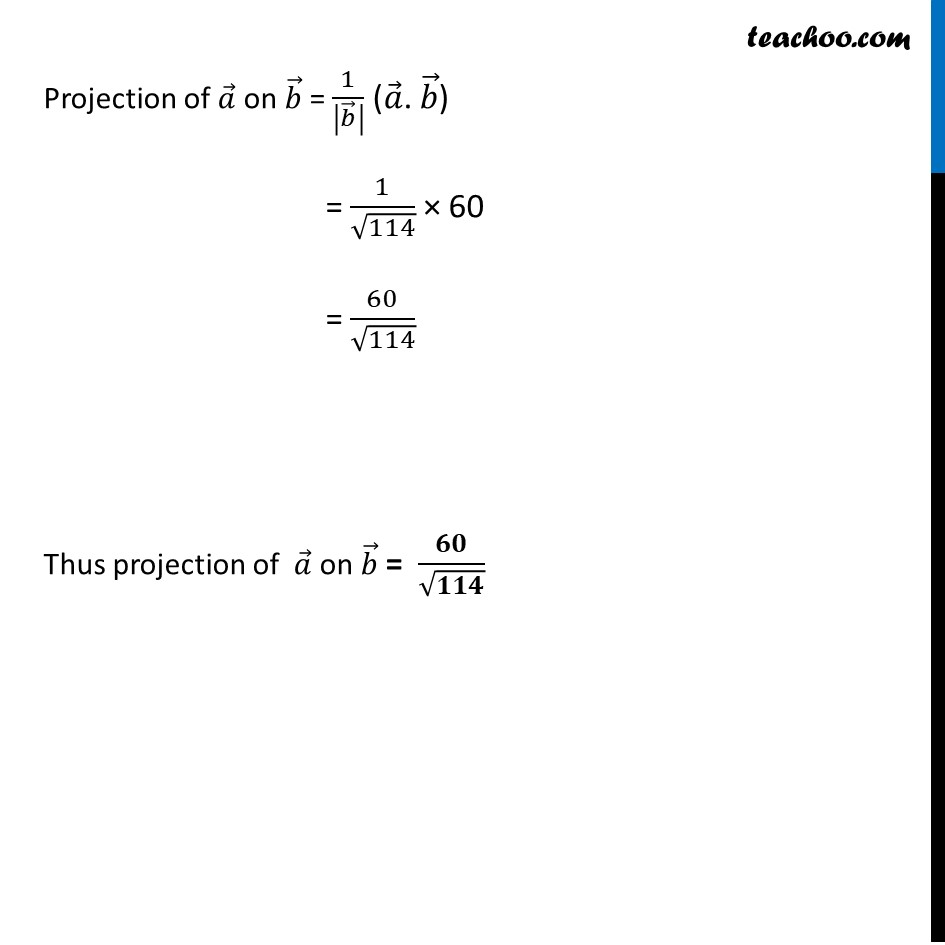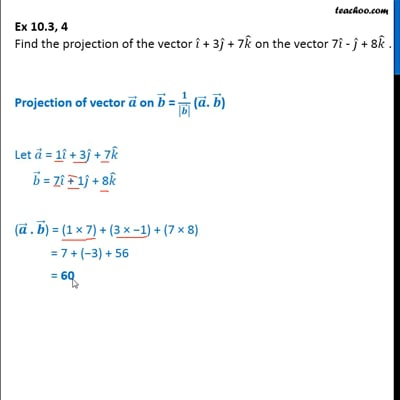Ex 10.3

Chapter 10 Class 12 Vector Algebra
Serial order wiseThis video is only available for Teachoo black users

Introducing your new favourite teacher - Teachoo Black, at only ₹83 per month

### Transcript

Ex 10.3, 4 Find the projection of the vector 𝑖 ̂ + 3𝑗 ̂ + 7𝑘 ̂ on the vector 7𝑖 ̂ - 𝑗 ̂ + 8𝑘 ̂ . Projection of vector 𝒂 ⃗ on 𝒃 ⃗ = 𝟏/|𝒃 ⃗ | (𝒂 ⃗. 𝒃 ⃗) Let 𝑎 ⃗ = 1𝑖 ̂ + 3𝑗 ̂ + 7𝑘 ̂ 𝑏 ⃗ = 7𝑖 ̂ – 1𝑗 ̂ + 8𝑘 ̂ (𝒂 ⃗ . 𝒃 ⃗) = (1 × 7) + (3 × −1) + (7 × 8) = 7 + (−3) + 56 = 60 Magnitude of 𝑏 ⃗ = √(72+(−1)2+82) |𝑏 ⃗ | = √(49+1+64) = √𝟏𝟏𝟒 Projection of 𝑎 ⃗ on 𝑏 ⃗ = 1/|𝑏 ⃗ | (𝑎 ⃗. 𝑏 ⃗) = 1/√114 × 60 = 60/√114 Thus projection of 𝑎 ⃗ on 𝑏 ⃗ = 𝟔𝟎/√𝟏𝟏𝟒